PSAT Math : Integers

Example Questions

← Previous 1 3 4 5 6 7 8 9 18 19

Example Question #1 : How To Find Absolute Value

If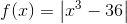, what is the value of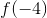?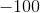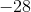Explanation:

Substitute – 4 in for x. Remember that when a negative number is raised to the third power, it is negative. -  = – 64. – 64 – 36 = – 100. Since you are asked to take the absolute value of – 100 the final value of f(-4) = 100. The absolute value of any number is positive.

Example Question #1 : How To Find Absolute Value

For which of the following functions below does f(x) = |f(x)| for every value of x within its domain?

f(x) = 2x + 3

f(x) = x2 – 2x

f(x) = x4 + x

f(x) = x4 + (1 – x)2

f(x) = x2 – 9

f(x) = x4 + (1 – x)2

Explanation:

When we take the absolute value of a function, any negative values get changed into positive values. Essentially, |f(x)| will take all of the negative values of f(x) and reflect them across the x-axis. However, any values of f(x) that are positive or equal to zero will not be changed, because the absolute value of a positive number (or zero) is still the same number.

If we can show that f(x) has negative values, then |f(x)| will be different from f(x) at some points, because its negative values will be changed to positive values. In other words, our answer will consist of the function that never has negative values.

Let's look at f(x) = 2x + 3. Obviously, this equation of a line will have negative values. For example, where x = –4, f(–4) = 2(–4) + 3 = –5, which is negative. Thus, f(x) has negative values, and if we were to graph |f(x)|, the result would be different from f(x). Therefore, f(x) = 2x + 3 isn't the correct answer.

Next, let's look at f(x) = x2 – 9. If we let x = 1, then f(1) = 1 – 9 = –8, which is negative. Thus |f(x)| will not be the same as f(x), and we can eliminate this choice as well.

Now, let's examine f(x) = x2 – 2x. We know that x2 by itself can never be negative. However, if x2 is really small, then adding –2x could make it negative. Therefore, let's evaluate f(x) when x is a fractional value such as 1/2. f(1/2) = 1/4 – 1 = –3/4, which is negative. Thus, there are some values on f(x) that are negative, so we can eliminate this function.

Next, let's examine f(x) = x4 + x. In general, any number taken to an even-numbered power must always be non-negative. Therefore, x4 cannot be negative, because if we multiplied a negative number by itself four times, the result would be positive. However, the x term could be negative. If we let x be a small negative fraction, then x4 would be close to zero, and we would be left with x, which is negative. For example, let's find f(x) when x = –1/2. f(–1/2) = (–1/2)+ (–1/2) = (1/16) – (1/2) = –7/16, which is negative. Thus, |f(x)| is not always the same as f(x).

By process of elimination, the answer is f(x) = x4 + (1 – x)2 . This makes sense because x4 can't be negative, and because (1 – x)can't be negative. No matter what we subtract from one, when we square the final result, we can't get a negative number. And if we add x4 and (1 – x)2, the result will also be non-negative, because adding two non-negative numbers always produces a non-negative result. Therefore, f(x) = x4 + (1 – x)will not have any negative values, and |f(x)| will be the same as f(x) for all values of x

The answer is f(x) = x4 + (1 – x)2 .

Example Question #2 : How To Find Absolute Value

Letandboth be negative numbers such that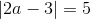and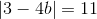. What is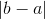?Explanation:

We need to solve the two equations |2a – 3| = 5 and |3 – 4b| = 11, in order to determine the possible values of a and b. When solving equations involving absolute values, we must remember to consider both the positive and negative cases. For example, if |x| = 4, then x can be either 4 or –4.

Let's look at |2a – 3| = 5. The two equations we need to solve are 2a – 3 = 5 and 2a – 3 = –5.

2a – 3 = 5 or 2a – 3 = –5

2a = 8 or 2a = –2

Divide by 2.

a = 4 or a = –1

Therefore, the two possible values for a are 4 and –1. However, the problem states that both a and b are negative. Thus, a must equal –1.

Let's now find the values of b.

3 – 4b = 11 or 3 – 4b = –11

Subtract 3 from both sides.

–4b = 8 or –4b = –14

Divide by –4.

b = –2 or b = 7/2

Since b must also be negative, b must equal –2.

We have determined that a is –1 and b is –2. The original question asks us to find |b – a|.

|b – a| = |–2 – (–1)| = | –2 + 1 | = |–1| = 1.

Example Question #1 : How To Find Absolute Value

Evaluate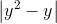if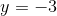.Explanation:

Remember that the absolute value of a number is the positive value of that number. It is important to know what absolute value represents: the distance of any given number from the number 0. For that reason, absolute value cannot be negative, and we can eliminateandfrom our answer choices

To solve, substituteinto the equation, taking extra care to correctly calculate the negative numbers: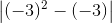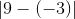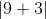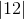Example Question #3 : How To Find Absolute Value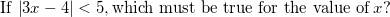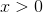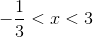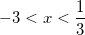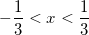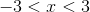Explanation: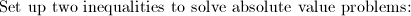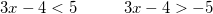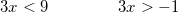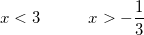Example Question #3 : How To Find Absolute Value

How many of these statements are correct?

I)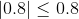II)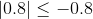III)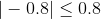IV)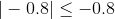Two

One

Four

None

Three

Two

Explanation:

The absolute value of a nonnegative number is the number itself; the absolute value of a negative number can be obtained by removing the negative symbol. Therefore,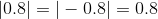.

The four statements can be rewritten as:

Both I and III)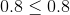- This is true.

Both II and IV)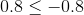- This is false.

The correct response is two.

Example Question #1 : Number Line

If 0 < n < 1, then which of the following is the smallest?

–1/n3

1/n2

n2

–1/n

n3 – 1

–1/n3

Explanation:

First, it will help us to determine which of the answer choices are positive and which are negative.

Because n is positive, we know that n2 is positive, because any number squared is positive. Similarly, 1/n2 is also positive.

Let's look at the answer choice –1/n. This must be negative, because a negative number divided by a positive one will give us a negative number. Similarly, –1/n3 will also be negative.

The last choice is n3 – 1. We are told that 0 < n < 1. Because n is a positive value less than one, we know that 0 < n3 < n2 < n < 1. In other words, n3 will be a small positive value, but it will still be less than one. Thus, because n3 < 1, if we subtract 1 from both sides, we see that n3 – 1 < 0. Therefore, n3 - 1 is a negative value.

All negative numbers are less than positive numbers. Thus, we can eliminate n2 and 1/n2, which are both positive. We are left with –1/n, –1/n3, and n3 – 1.

Let us compare –1/n and –1/n3. First, let us assume that –1/n < –1/n3.

–1/n < –1/n3

Multiply both sides by n3. We don't need to switch the signs because n3 is positive.

–n< –1

Multiply both sides by –1.

n2 > 1.

We know that n2 will only be bigger than 1 when n > 1 or if n < –1. But we know that 0 < n < 1, so –1/n is not less than –1/n3. Therefore, –1/nmust be smaller.

Finally, let's compare –1/n3 and n3 – 1. Let us assume that –1/n3 < n3 – 1.

–1/n3 < n3 – 1

Multiply both sides by n3.

–1 < n6 – n3

n6 – n3 + 1 > 0. If this inequality is true, then it will be true that –1/n3 is the smallest number.

Here it will be helpful to try some values for n. Let's pick n = 1/2 and see what happens. It will help to use our calculator.

(1/2)6 – (1/2)3 + 1 = 0.891 > 0.

Therefore, we suspect that because n6 – n3 + 1 > 0, –1/nis indeed the smallest number. We can verify this by trying more values of n.

Example Question #1 : Number Lines And Absolute Value

What property of arithmetic is demonstrated here?

If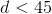and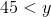, then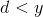.

Inverse

Associative

Transitive

Commutative

Symmetric

Transitive

Explanation:

The symbols express the idea that if a number is less than a second number, which is less than a third, then the first number is less than the third. This is the transitive property of inequality.

Example Question #1 : How To Graph An Inequality With A Number Line

On a real number line, x1 = -4 and x2 = 14. What is the distance between these two points?

-18

10

18

4

18

Explanation:

The distance between two points is always positive. We calculate lx2 - x1l, which will give us the distance between the points.

|14- (-4)| = |14+4| = |18| = 18

Example Question #2 : How To Graph An Inequality With A Number Line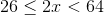Which of the following is a graph for the values ofdefined by the inequality stated above?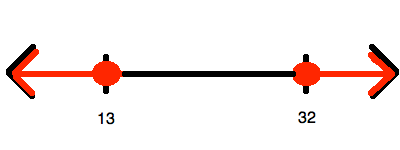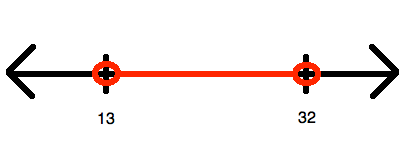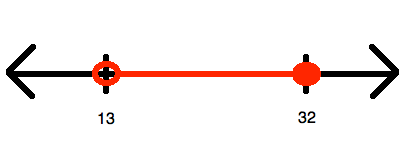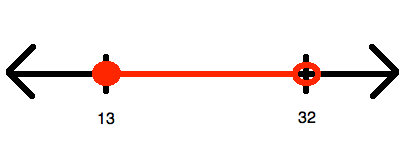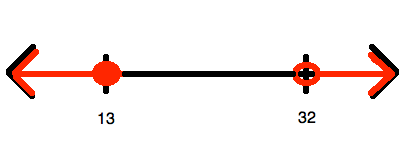Explanation:

To begin, you must simplify so that you "isolate", (i.e. at least eliminate any coefficients from it). To do this, divide all of the members of the inequality by: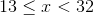Now, this inequality represents all of the numbers between 13 and 32.  However, it does include(hence, getting a closed circle for that value) and does not include(hence, getting an open circle for that value). Therefore, it looks like:← Previous 1 3 4 5 6 7 8 9 18 19